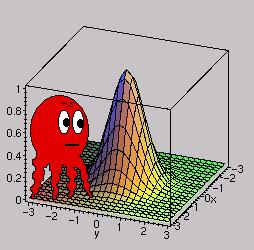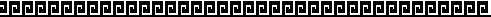Financial mathematics

In the last years I got more and more interested in questions of financial mathematics like Value at risk, Portfolio-optimization or Option-pricing. Here are some of my works. They are no high-end real life applications - Excel is not the tool for such tasks - but they show the mathematical idea.

• Pricing of an american plain Vanilla option. A little Excel-Sheet which calculates the price of american Put and Call-Options on a stock. I used the binomial-tree modell, also know as the CRR-Modell
• Calculation of a bond price. Another Excel-Sheet which calculates the price of an "regular" bond from the expected cash-flows and a given yield-curve. Nothing special, but it gives you a feeling how cash-flows, yield-curve and bond-prices interact.
• Monte Carlo Value at Risk. An Excel-Sheet that calculates the VAR for a portfolio of three risk factors. The number of simulations is limited to 10000. The empirical distrubutions of the portfolio values is provided. On a second sheet the covariance matrix of rel. returns can be estimated from historical prices. The calculation follow the idea of RiskMetrics.[ Startpage ] --- [ gästebuch ] --- [ sitemap ] --- up to date: 04-08-2003